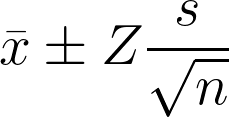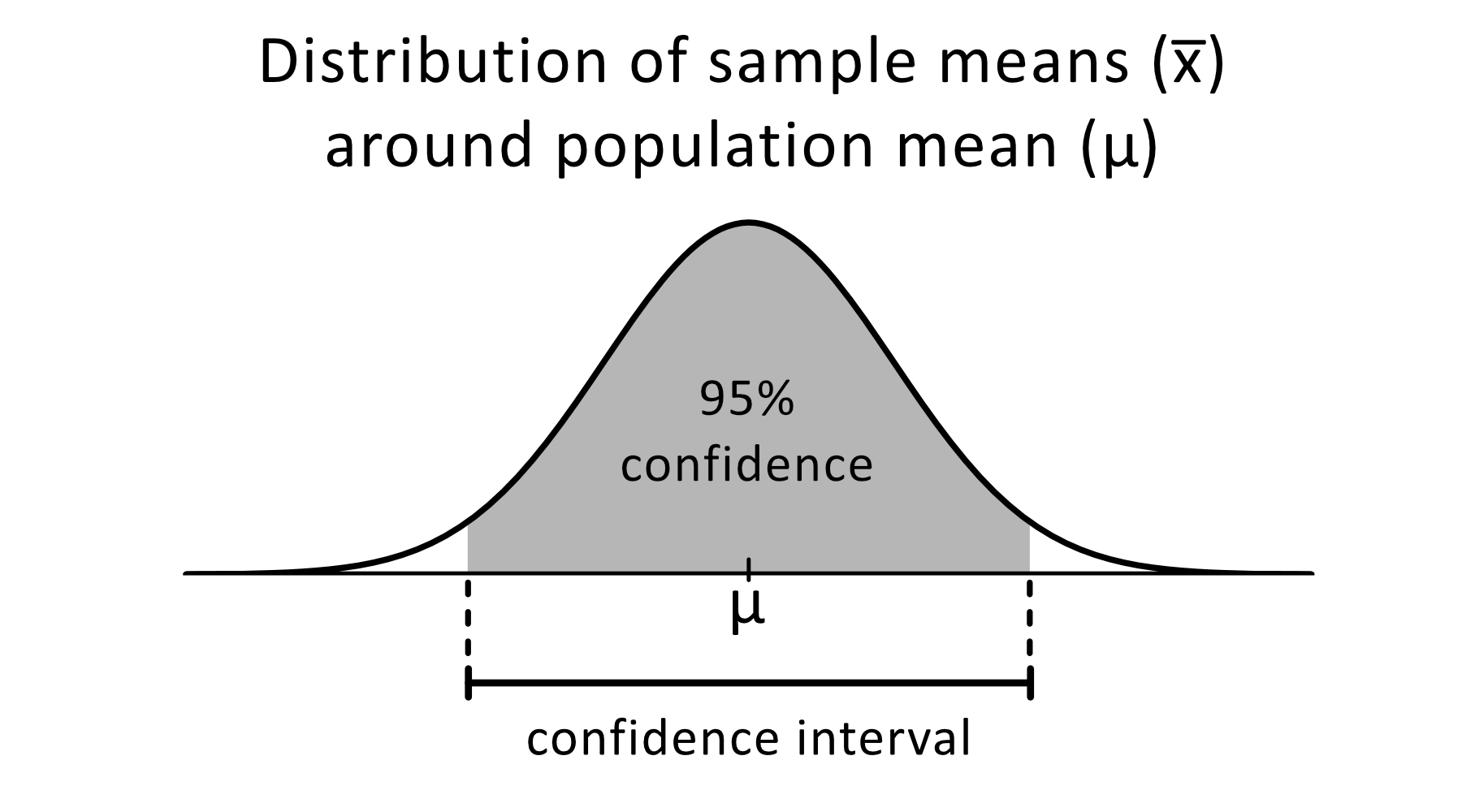# 95% Confidence Interval Calculator

Created by Aleksandra Zając, MD
Last updated: Feb 02, 2023

If you want to solve some confidence interval problems, you're in the right place. Our 95% confidence interval calculator will help you calculate this confidence interval and provide you with the essential knowledge! Read on to learn:

• What is the 95% confidence interval formula;
• What is the interpretation of the 95% confidence interval (or any chosen one, to be honest); and
• What is the p-value for the 95 percent confidence interval?

## 95% confidence interval - formula and interpretation

To calculate a 95% confidence interval, we first need to calculate the standard error. We can use the formula:

$SE=\frac{σ}{\sqrt{n}}$

where:

• $SE$ - the standard error;
• $σ$ - the standard deviation; and
• $n$ - number of measurements (the size of the sample).

Now let's estimate the margin of error.

$ME = SE × Z(0.95)$
• $ME$ - margin of error;
• $Z(0.95)$ - z-score for 95% confidence level; you'll find this value in the statistical tables.

The only thing left is to count the lower and upper bounds of our confidence interval. To do this, we will add and subtract the margin of error to the mean (average) - $μ$.

$\text{upper}\ \text{bound} = μ + ME$
$\text{lower}\ \text{bound} = μ - ME$

## Interpretation of the 95% confidence interval

How should you understand the results? Let's imagine George wants to calculate the mean height of his family members. Some relatives live very far, and George cannot measure them all. But he managed to get 30 measurements, and the average height of those measurements was 172 cm (5 ft 7 in). He found out, that the 95% confidence interval range is 161-183 cm (5 ft 3 in - 6 ft). Based on those measurements, he can be 95% sure that any family member's height falls between 162-183 cm.

## Using 95% confidence interval calculator

To use our tool:

1. Look at the calculator panel on the left side of the screen.
2. First, fill in the sample mean (average) - x̅.
3. Now, fill in the standard deviation (s).
4. Input the sample size (n).
5. As it is the 95 percent confidence interval calculator, the confidence interval value is already there. But remember - you might change it any time.
6. The Z-score row will change accordingly to your chosen confidence interval.
7. Enjoy the results! You might now see the margin of error, the 95% confidence interval bounds, but also a chart displaying the data.

## Confidence interval calculators

Check out the rest of our confidence interval tools.

## FAQ

### How do I calculate a 95% confidence interval?

To count the 95% confidence interval:

1. First, calculate the standard error (SE) and the margin of error (ME).
SE = σ/√n
ME = SE × Z(0.95)
where σ is the standard deviation, n - sample size, Z(0.95) - z-score for 95% confidence interval.
2. Then determine the confidence interval range, using ME and μ - the calculated average (mean).
upper bound = μ + ME
lower bound = μ - ME

### What is the p-value at 95 percent confidence interval?

The uncorrected p-value at 95% confidence interval is 0.05.

Aleksandra Zając, MDSample mean (x̅)
Standard deviation (s)
Sample size (n)
Confidence level
%
or Z-score (Z)
σ95% of samples contain the population mean (μ) within the confidence interval x̅ ± E.
Confidence interval
Lower bound
Upper bound
Margin of error (E)
People also viewed…

### Dot plot

Make your own dot plots with this dot plot calculator!

### Humans vs vampires

Vampire apocalypse calculator shows what would happen if vampires were among us using the predator - prey model.

### Meat footprint

Check out the impact meat has on the environment and your health.

### Wilcoxon rank-sum test

The Wilcoxon rank-sum test calculator allows you to perform the famous non-parametric test based on the ranks of observations. Both exact and approximated versions are available, along with corrections for ties and continuity!